# B008 – Calorific Value

## Description

This block calculates the uncertainty in the calorific value of a gas composition as determined by the selected calculation method.

## Options

#### Reference

• AGA 5
• ASTM D3588
• ISO 6976
• API MPMS Ch 14.5/GPA 2172

This option is used to select which reference Calorific Value will be calculated according to.

#### Calorific value

• Volume based
• Mass based

This option determines which type of calorific value is to be used.

Note: this option is available for all references except API MPMS Ch 14.5/GPA 2172.

### AGA 5

If AGA 5 is selected the following options will be available to the user: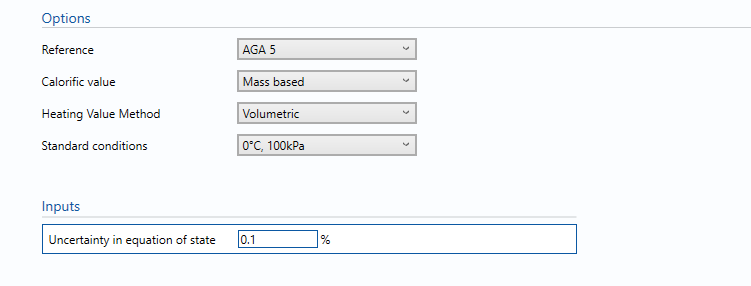#### Heating Value Method

• Molar
• Volumetric

This option is selected to use molar or volumetric heating values from the physical data tables.

#### Standard Temperature

• 0°C
• 15°C
• 20°C
• 25°C
• 60°F

This option allows the user to select the standard temperature at which the physical properties are referenced to.

Note: This option is available if Molar is selected from the Heating Value Method option.

#### Standard Conditions

• 0°C/100kPa
• 15°C/101.325kPa
• 20°C/98.0665kPa
• 59°F/14.696psi
• 60°F/14.4psi
• 60°F/14.65psi
• 60°F/14.696psi
• 60°F/14.73psi
• 60°F/15.025psi

This option allows the user to select the standard conditions at which the physical properties are referenced to.

Note: This option is available if Volumetric is selected from the Heating Value Method option.

### Inputs

U(EOS)

Uncertainty in the equation of state. Guidelines on determining this value can be found in the relevant standard.

### ASTM D3588

If ASTM D3588 is selected the following options will be available to the user: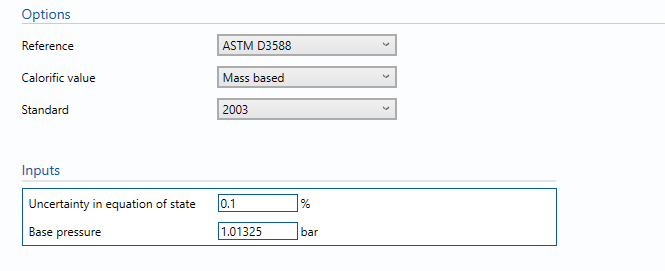#### Standard

• 1989
• 2000
• 2003
• 2009

This option determines which version of the GPA 2145 standard data tables is used for the property data required for the ASTM D3588 calculation of Calorific Value.

### Inputs

U(EOS)

Uncertainty in the equation of state. Guidelines on determining this value can be found in the relevant standard.

Base Pressure

Enter the absolute pressure at standard conditions.

### ISO 6976

If ISO 6976 is selected the following options will be available to the user: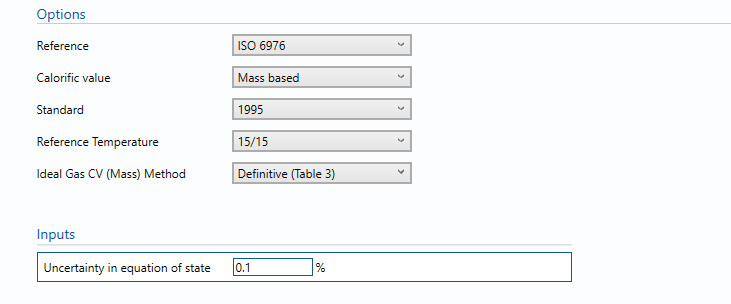#### Standard

• 1983
• 1989
• 1995

This option determines which version of ISO 6976 will be used for the calculation of Calorific Value.

#### Reference Temperature (Combustion/Metering)

• 25/0
• 15/0
• 0/0
• 15/15
• 25/20 (only available if 1989 or 1995 is selected)
• 20/20 (only available if 1995 is selected)
• 25/15 (only available if 1995 is selected)

This option is used to select the combustion and metering temperatures at which the calorific value is calculated for.

Note: temperatures are in degrees Celcius.

#### Ideal Gas Calorific Value (Mass) method

• Definitive (Table 3)
• Alternative (Table 4)

This option is used to select whether the ideal volumetric calorific value is obtained using Table 3 molar calorific values or Table 4 round mass calorific values. It is only available if the 1995 standard is selected.

#### Ideal Gas Calorific Value (Volumetric) method

• Definitive (Table 3)
• Alternative (Table 5)

This option is used to select whether the ideal volumetric calorific value is obtained using Table 3 molar calorific values or Table 5 round volumetric calorific values. It is only available if the 1995 standard is selected.

### Inputs

U(EOS)

Uncertainty in the equation of state. Guidelines on determining this value can be found in the relevant standard.

### API MPMS Ch 14.5/GPA 2172

If API MPMS Chapter 14.5 / GPA 2172 is selected the following options will be available to the user: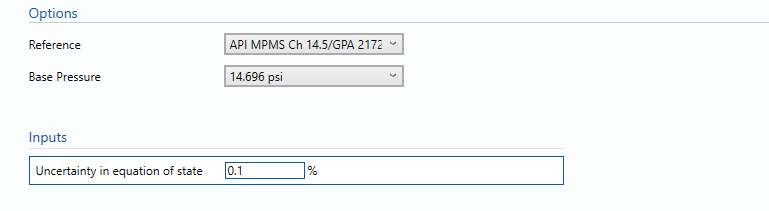#### Base pressure

• 14.696psi
• 14.73psi
• 14.7345psi

This option is used to select the base pressure at which the calculation is referred to.

### Inputs

U(EOS)

Uncertainty in the equation of state. Guidelines on determining this value can be found in the relevant standard.

## Calculations

The gas calorific value is calculated using the selected standard according to the option selected.The gas composition and its individual uncertainties are taken from the block B004 – Composition.

### Uncertainty Budget

The uncertainty calculation is detailed in the uncertainty budget table giving a break down of how the overall uncertainty is calculated. The values input into the uncertainty budget are taken from calculations from other blocks along with the uncertainty in the equation of state as input in this block.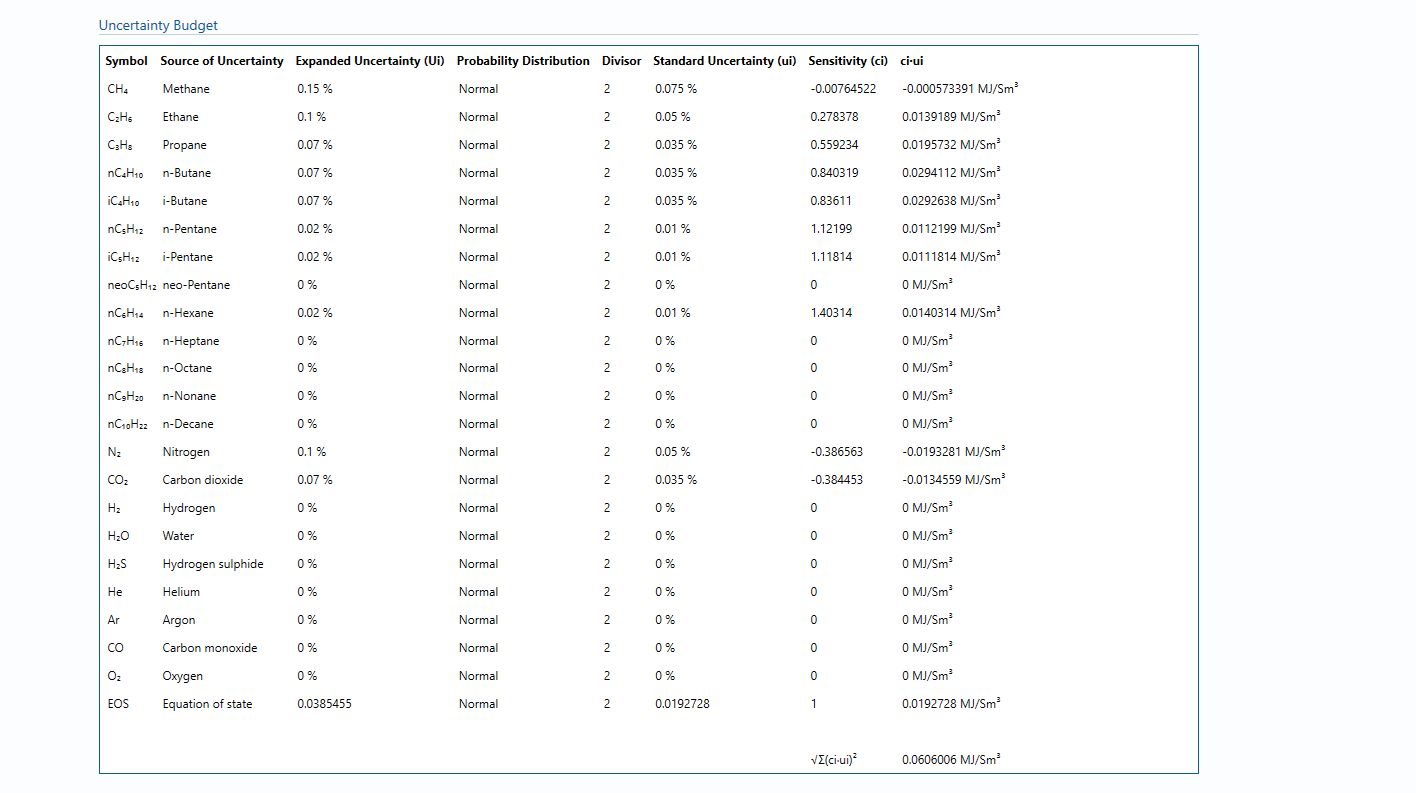Uncertainties associated with these values are taken in as the Expanded Uncertainties. The Standard Uncertainty is calculated from dividing the Expanded Uncertainty by the coverage factor which is determined by the probability distribution that best suits that uncertainty component.

The standard uncertainty is then multiplied by the sensitivity value then squared. This is done for each parameter that contributes to the overall uncertainty in the calorific value. The Standard Uncertainty the calorific value the square root of the sum of each component variance as shown in the following equation: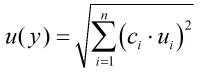#### Calculated Uncertainty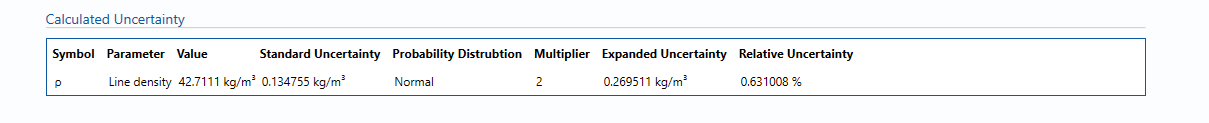The Expanded Uncertainty is the Standard Uncertainty multiplied by the coverage factor (k). The coverage factor is defaulted to k = 2 (equivalent to a confidence level of approximately 95%).

The Relative Uncertainty is the Expanded Uncertainty divided by the gas standard density value.

### Standards

ISO 5168:2005 – Measurement of fluid flow – Procedures for the evaluation of uncertainties

ISO Guide 98-3 – Uncertainty of measurement – Part 3: Guide to the expression of uncertainty in measurement (GUM:1995)

AGA Report No. 5 – Natural Gas Energy Measurement (March 2009)

ISO 6976:1983 – Natural gas – Calculation of calorific value, density and relative density

ISO 6976:1989 – Natural gas – Calculation of calorific value, density and relative density

ISO 6976:1995 – Natural gas – Calculation of calorific values, density, relative density and Wobbe index from composition

ASTM D3588-98: Standard Practice for Calculating Heat Value, Compressibility Factor and Relative Density of Gaseous Fuels (1998)

GPA 2145 Table of Physical Properties for Hydrocarbons and Other Compounds of Interest to the Natural Gas Industry (1989, 2000, 2003, 2009)

GPA 2172-09 – Calculation of Gross Heating Value, Relative Density, Compressibility, and Theoretical Hydrocarbon Liquid Content for Natural Gas Mixtures for Custody Transfer (2009)

API Manual of Petroleum Measurement Standards Chapter 14.5 – Calculation of Gross Heating Value, Relative Density, Compressibility, and Theoretical Hydrocarbon Liquid Content for Natural Gas Mixtures for Custody Transfer (2009)

### KCCL Calculations

C103 – GHV, RD and Compressibility

C111 – Calorific Value and Relative Density – ISO 6976

C127 – AGA 5:2009 – Natural Gas Energy Measurement

C263 – ASTM D3588/GPA 2145 – Calorific Value and Relative Density

### Uncertainty Blocks

B004 – Composition

Back to Uncertainty Modules